## 1. Overview

There are various logical approaches in computer science, and fuzzy logic is one of them.

In this tutorial, we’ll take a look at what this approach means and how it works. Then, we’ll use an example to understand fuzzy logic in a better way.

## 2. Fuzzy Logic

Let’s have a look at what is fuzzy logic and why we use it.

### 2.1. What Is Fuzzy Logic?

Lotfi Zadeh developed fuzzy logic after observing that, unlike computers, people have a different range of possibilities between YES and NO. Fuzzy Logic (FL) is a method of reasoning that resembles human reasoning. This approach is similar to how humans perform decision-making. It involves all intermediate possibilities between YES and NO.

Let’s answer the question “Is it cold?” using the boolean and fuzzy logic:

• Boolean logic: the answer could be either TRUE or FALSE. It only takes the value to be asor, which is equivalent to a human being’s YES or NO:• Fuzzy logic: If we ask the same question “Is it cold?”, we’ll get different answers like “very much cold”, “it’s a little cold”, or “very less cold”. So, we’ll also get the intermediate possibilities between YES or NO: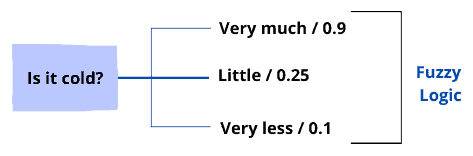### 2.2. Why Do We Use Fuzzy Logic?

In general, we employ the fuzzy logic system for commercial and practical reasons:

• It controls machines and consumer products
• If not accurate reasoning (YES or NO), it at least provides acceptable reasoning (it might be or it can happen)
• This helps engineers deal with uncertainty. So, in case we are unsure if the answer can be YES or NO, we can find a middle path.

## 3. Architecture and Membership Function

The architecture of fuzzy logic consists of four main components: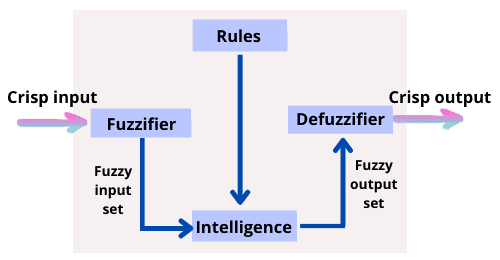### 3.1. Rules

It includes all the rules and if-then conditions proposed by experts to control the decision-making system. The current update to the fuzzy approach gives various practical methods for designing and tuning fuzzy controllers. These advancements typically reduce the number of fuzzy rules.

### 3.2. Fuzzifier

Here’s where the fuzzification takes place. This step converts the inputs or the crisp numbers into fuzzy sets. So, we can measure the crips inputs by sensors and pass them into the control system for further processing. The fuzzification split the input intosteps: LP (Large Positive), MP (Medium Positive), S (Small), MN (Medium Negative), and LN (Large Negative).

### 3.3. Intelligence

Provides the degree of match between the fuzzy input and the rules. So, according to the input field, it will decide the rules that are to be fired. The control actions will be formed by combining the fired rules. So, from here we’ll get the fuzzy output set.

### 3.4. Defuzzifier

It is used to transform the fuzzy sets produced by the intelligence engine into a crisp value. There are numerous defuzzification methods available, and the most appropriate one is employed in conjunction with a certain expert system to decrease the error.

### 3.5. Membership Function

The membership function is a graph that explains how each point in the input space is assigned a membership value ranging fromto. It allows us to quantify linguistic words and graphically display a fuzzy set. A membership function for a fuzzy seton the universe of discourseis defined as.

The membership function quantifies the degree of the membership of the element into the fuzzy set. The x-axis represents the universe of discourse whereas the y-axis represents the degrees of membership in. There can be multiple membership functions applicable to fuzzify a numerical value:

• Singleton fuzzifier
• Gaussian fuzzifier
• Trapezoidal or triangular fuzzifier

Here are the membership functions for LP (Large Positive), MP (Medium Positive), S (Small), MN (Medium Negative), and LN (Large Negative):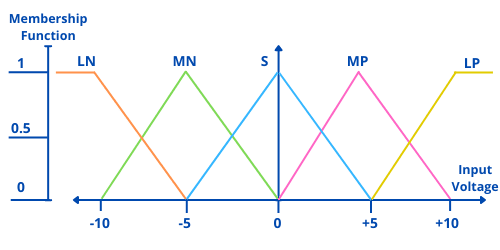The x-axis represents the membership function whereas the y-axis represents the input voltage. The triangular membership function shapes are common among various other membership function shapes. The input to different level fuzzifier (LN, MN, S, MP, LP) ranges fromvolts tovolts. Hence, the corresponding output also changes. So, based on these volts the value for the membership function will also change.

## 4. Fuzzy Logic vs Probability

The fuzzy logic is often confused with probability. Let’s then take a look at the differences between them:Let’s go through the applications of fuzzy logic before diving into its benefits and drawbacks.

### 5.1. Applications of Fuzzy Logic

Fuzzy logic is used in various fields, such as automotive systems, environment control, and domestic goods. Here are some of the common applications:

• The aerospace field for altitude control of spacecraft and satellite
• Control the speed and traffic in the automotive systems
• Support systems and personal evaluation concerning decision making in large company business
• This controls the pH, drying, and chemical distillation process in the chemical industry
• Natural language processing and various intensive applications in Artificial Intelligence
• Fuzzy Logic mimics how humans make decisions, but much faster. As a result, it can be used with Neural Networks

Same as any approach, fuzzy logic has its benefits and drawbacks. Let’s then take a look at the benefits and drawbacks of the fuzzy logic in artificial intelligence:## 6. Example of Fuzzy Logic in AI

Let’s take a simple example to understand the fuzzy logic in a better way. The design of a fuzzy system starts with a set of membership functions for each input and a set for each output. A set of rules is then applied to the membership functions to yield a crisp output value.

Let’s take the example of process control. Before designing we need to understand what we are trying to control, and what data inputs we have that allow us to measure that control? Answering that will allows us to define the linguistic values for the system.

Let’s dive directly into the example for the fuzzy logic. For process control, we have temperature and fan speed. In other words, we are trying to control the speed based on the temperature. Now that we have answered the two most important questions, other things will fall in place.

### 6.1. Membership Functions

We have the temperature as the input and the fan speed as the output. So, here we have created a set of membership functions for each input: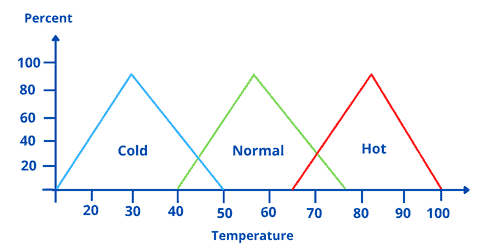Then, we’ll create a membership function for each of these three sets of temperatures. So, forandaxis we have temperature and percent. We have three fuzzy sets: cold, normal or warm, and hot. In the next step, we’ll use the three fuzzy sets for the output. We have slow, medium, and fast: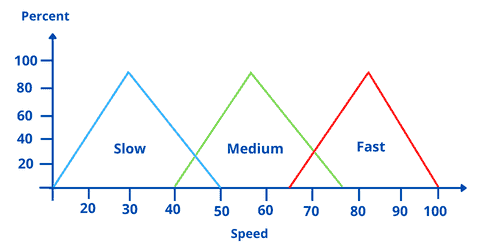So, for the input we have temperature and we have taken the speed as the output. That’s the fan speed.

### 6.2. Rules

We have defined the membership functions. Let’s now move forward to create the rules for these functions. These rules define how the membership functions will be applied to the final system. So, let’s create three rules for our system:

• Rule 1: If the temperature is hot then the speed will be fast
• Rule 2: If the temperature is warm then the speed will be medium
• Rule 3: If the temperature is cold then the speed will be slow

According to these three rules, we are comparing the input with the output. Then, we calculate the speed based on the temperature. Thus, we apply these rules to the membership functions to produce the crisp output value to drive the system: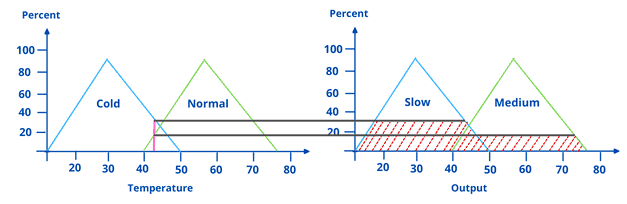For an input value of arounddegrees, we intersect the membership function. So, we are applying two rules as the intersection occurs on both functions. We can extend the intersection points to the output function to produce an intersecting point.

To obtain the best crisp output, the defuzzification procedure is applied to the fuzzy output set. There are numerous defuzzification methods, such as the centroid method, center of largest area method, and first maxima method.

## 7. Conclusion

In this tutorial, we’ve discussed the basic concept of fuzzy logic using an example to unlock its mechanism. We’ve also gone over the principle of fuzzy logic in artificial intelligence and how it works.